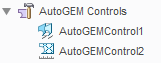cancel
Showing results for
Did you mean:
cancel
Showing results for
Did you mean:

SOLVED
Highlighted

Acceleration on Body

Hello,

Is it possible in Simulate investigate the behaviour of body fixed on slide carriage with acceleration force?

Thank you

Tags (5)
1 ACCEPTED SOLUTION

Accepted Solutions

example att.

10 REPLIES 10

example att.

Re: Acceleration on Body

When use "Gravity Force", it is necessary that the WCS is in centroid G of the mass;

if not, I need to create a new coordinate system with X = Xg, Y = Yg, Z = Zg, correct?

yes

Re: Acceleration on Body

If I would like to consider the own weight (z = - 9.806 [m/s2]) of the 3D body that is in Simulate, I need add a Ideal mass in the Centroid G?

Re: Acceleration on Body

gravitation works on all bodies

Re: Acceleration on Body

But, for every surface I can know the value of force on it?

example: force1

Re: Acceleration on Body

what is the purpose of these?Thank you

Re: Acceleration on Body

What does it mean this?

Warning: Resultant measure Forza_10
may not be evaluated correctly because there are
multi-point constraints (MPCs) near the resultant measure.
MPCs may occur in models with mixed or
incompatible meshes, links, fasteners, or
user coordinate system (UCS) constraints.

I found this topic

https://www.ptc.com/en/support/article?n=CS95825&source=snippet

So, also in your example the surfaces of measure and of the rigid link are the same...